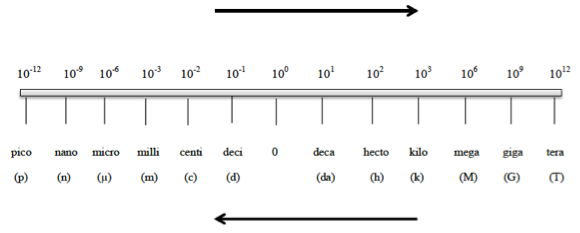# Problem: Use scientific notation to express each of the following quantities with only the base units (no prefix multipliers).5.6 ns

###### FREE Expert Solution

Metric Prefixes are “labels” that can be placed in front of base units. Whenever using the metric prefix chart, remember that “1” is associated with the metric prefixConversion factor:

• 1 ns = 10-9 s (base unit)###### Problem Details
Use scientific notation to express each of the following quantities with only the base units (no prefix multipliers).

5.6 ns